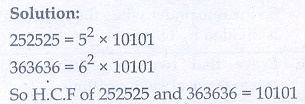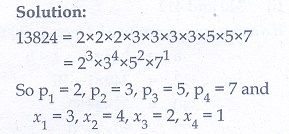Home | | Maths 10th Std | Exercise 2.2: Fundamental Theorem of Arithmetic

# Exercise 2.2: Fundamental Theorem of Arithmetic

Maths Book back answers and solution for Exercise questions - Mathematics : Numbers and Sequences: Fundamental Theorem of Arithmetic: Exercise Problem Questions with Answer

Exercise 2.2

1. For what values of natural number n, 4n can end with the digit 6?2. If mare natural numbers, for what values of m, does 2n  ×5m ends in 5?3. Find the HCF of 252525 and 363636.4. If 13824 = 2a × 3b then find and b.5. If  p1x1  × p2x2  × p3x3  × p4x4   = 113400  where  p1 p2 p3 p4 are primes in ascending order and x1x2x3x4 are integers, find the value of p1,p2p3p4 and x1x2x3x4.6. Find the LCM and HCF of 408 and 170 by applying the fundamental theorem of arithmetic.7. Find the greatest number consisting of 6 digits which is exactly divisible by 24,15,36?.8. What is the smallest number that when divided by three numbers such as 35, 56 and91 leaves remainder 7 in each case?9. Find the least number that is divisible by the first ten natural numbers.1. Even number

2. No value

3. 10101

4. 9, 3

5. 2,3,5,7 and 3,4,2,1

6. 2040, 34

7. 999720

8. 3647 9. 2520

Tags : Problem Questions with Answer, Solution | Mathematics , 10th Mathematics : UNIT 2 : Numbers and Sequences
Study Material, Lecturing Notes, Assignment, Reference, Wiki description explanation, brief detail
10th Mathematics : UNIT 2 : Numbers and Sequences : Exercise 2.2: Fundamental Theorem of Arithmetic | Problem Questions with Answer, Solution | Mathematics